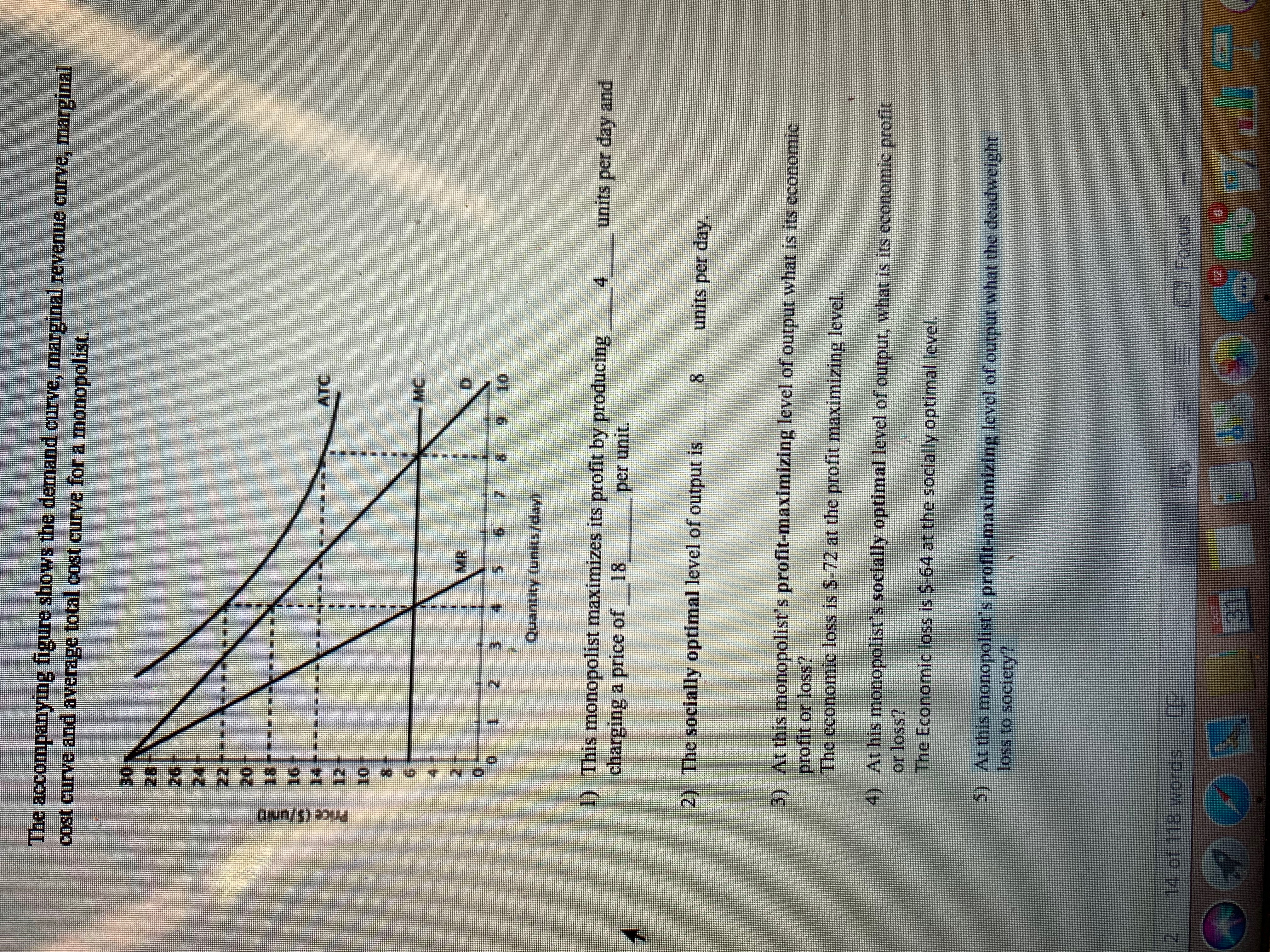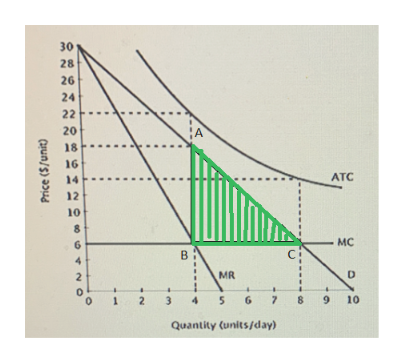# The accompanying figure shows the demand curve, marginal revenue curve, marginalcost eurve and average total cost curve for a monopolist.30282624222018-16ATC1210-MC4MR236910Quantity (units/day!1) This monopolist maximizes its profit by producingcharging a price of4units per day and18per unit2) The socially optimal level of output is8units per day.3) At this monopolist s profit-maximizing level of output what is its economicprofit or loss?The economie loss is \$-72 at the profit maximizing level.4) At his monopolist's socially optimal level of output, what is its economic profitor loss?The Economic loss is \$-64 at the socially optimal level.5) At this monopolist's profit-maximizing level of output what the deadweightloss to society!14 of 118 wordsFocus31பொ/து *uu

Question
356 views

5. At this monopolist’s profit-maximizing level of output what the deadweight loss to society?help_outlineImage TranscriptioncloseThe accompanying figure shows the demand curve, marginal revenue curve, marginal cost eurve and average total cost curve for a monopolist. 30 28 26 24 22 20 18- 16 ATC 12 10 -MC 4 MR 2 3 6 9 10 Quantity (units/day! 1) This monopolist maximizes its profit by producing charging a price of 4 units per day and 18 per unit 2) The socially optimal level of output is 8 units per day. 3) At this monopolist s profit-maximizing level of output what is its economic profit or loss? The economie loss is \$-72 at the profit maximizing level. 4) At his monopolist's socially optimal level of output, what is its economic profit or loss? The Economic loss is \$-64 at the socially optimal level. 5) At this monopolist's profit-maximizing level of output what the deadweight loss to society! 14 of 118 words Focus 31 பொ/து *uu fullscreen
check_circle

Step 1

5)
The deadweight loss is the economic efficiency lost whiles the profit maximization of the monopolist or any other form of imperfect competition in the market. The part of the consumer surplus which is not converted into the producer surplus and lost forever is the deadweight loss to the society.

Step 2

The socially optimum level of production is identified at the 8 units per day whereas the monopolist makes his output decision at the point of 4 units per day. Thus, there comes a triangle area above the marginal cost curve and below the demand curve which represents the deadweight loss in this case. The deadweight loss can be illustrated as follows:help_outlineImage Transcriptionclose30 28 26 24 22 20 18 16 АTC 14 12 10 8 6 в 4 MR 2 2 3 4 5 7 8 9 10 1 Quantity (unitsjday) MC fullscreen
Step 3

The deadweight loss can be calculated by calculating the area of the triangle ABC which can be done by multiplying the base with height and then with 0.5. The base value is calculated to be the difference between the socially optimal ...

### Want to see the full answer?

See Solution

#### Want to see this answer and more?

Solutions are written by subject experts who are available 24/7. Questions are typically answered within 1 hour.*

See Solution
*Response times may vary by subject and question.
Tagged in